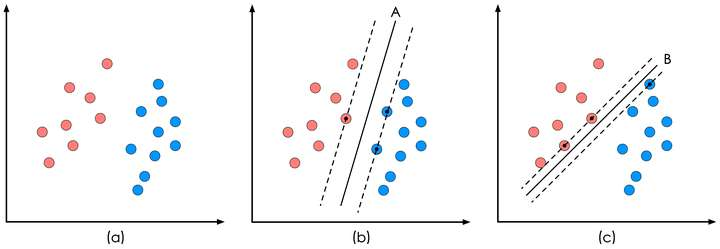### 支持向量机

（Support Vector Machine, SVM）的基本模型是在特征空间上找到最佳的 分离超平面使得训练集上正负样本间隔最大。

1、硬间隔支持向量机（线性可分支持向量机）：当训练数据线性可分时，可通过硬间隔最大化学得一个线性可分支持向量机。

1. 软间隔支持向量机：当训练数据近似线性可分时，可通过软间隔最大化学得一个线性支持向量机。
2. 非线性支持向量机：当训练数据线性不可分时，可通过核方法以及软间隔最大化学得一个非线性支持向量机。SVM算法认为图1中的分类器A在性能上优于分类器B，其依据是A的分类间隔比B要大

### 基于最大间隔分割数据

$w^{t}x+b$为分类函数SVM的目标函数：注：新目标函数约束条件：
alpha>=0,所有的aplha*lable=0

C>alpha>=0,所有的aplha*lable=0

SVM中的主要工作就是求解这些alpha。

SMO算法(序列最小优

SMO的工作原理是：每次循环中选择两个alpha进行优化处理，一旦找到一对合适的alpha，那么就增大其中一个，同时减小另一个。选择的alpha要满足在间隔边界之外的条件，而且还没有进行过区间化处理或者不再边界上。

### 核函数：Opencv代码：

#include <opencv2/core/core.hpp>
#include <opencv2/highgui/highgui.hpp>
#include <opencv2/ml/ml.hpp>

using namespace cv;

int main()
{
// Data for visual representation
int width = 512, height = 512;
Mat image = Mat::zeros(height, width, CV_8UC3);

// Set up training data
float labels = {1.0, -1.0, -1.0, -1.0,1.0};
Mat labelsMat(5, 1, CV_32FC1, labels);

float trainingData = { {501, 10}, {255, 10}, {501, 255}, {10, 501},{501,128} };
Mat trainingDataMat(5, 2, CV_32FC1, trainingData);

//设置支持向量机的参数
CvSVMParams params;
params.svm_type    = CvSVM::C_SVC;//SVM类型：使用C支持向量机
params.kernel_type = CvSVM::LINEAR;//核函数类型：线性
params.term_crit   = cvTermCriteria(CV_TERMCRIT_ITER, 100, 1e-6);//终止准则函数：当迭代次数达到最大值时终止

//训练SVM
//建立一个SVM类的实例
CvSVM SVM;
//训练模型，参数为：输入数据、响应、XX、XX、参数（前面设置过）
SVM.train(trainingDataMat, labelsMat, Mat(), Mat(), params);

Vec3b green(0,255,0), blue (255,0,0);
//显示判决域
for (int i = 0; i < image.rows; ++i)
for (int j = 0; j < image.cols; ++j)
{
Mat sampleMat = (Mat_<float>(1,2) << i,j);
//predict是用来预测的，参数为：样本、返回值类型（如果值为ture而且是一个2类问题则返回判决函数值，否则返回类标签）、
float response = SVM.predict(sampleMat);

if (response == 1)
image.at<Vec3b>(j, i)  = green;
else if (response == -1)
image.at<Vec3b>(j, i)  = blue;
}

//画出训练数据
int thickness = -1;
int lineType = 8;
circle( image, Point(501,  10), 5, Scalar(  0,   0,   0), thickness, lineType);//画圆
circle( image, Point(255,  10), 5, Scalar(255, 255, 255), thickness, lineType);
circle( image, Point(501, 255), 5, Scalar(255, 255, 255), thickness, lineType);
circle( image, Point( 10, 501), 5, Scalar(255, 255, 255), thickness, lineType);
circle(image, Point( 501, 128), 5, Scalar(0, 0, 0), thickness, lineType);

//显示支持向量
thickness = 2;
lineType  = 8;
//获取支持向量的个数
int c     = SVM.get_support_vector_count();

for (int i = 0; i < c; ++i)
{
//获取第i个支持向量
const float* v = SVM.get_support_vector(i);
//支持向量用到的样本点，用灰色进行标注
circle( image,  Point( (int) v, (int) v),   6,  Scalar(128, 128, 128), thickness, lineType);
}

imwrite("result.png", image);        // save the image

imshow("SVM Simple Example", image); // show it to the user
waitKey(0);

}